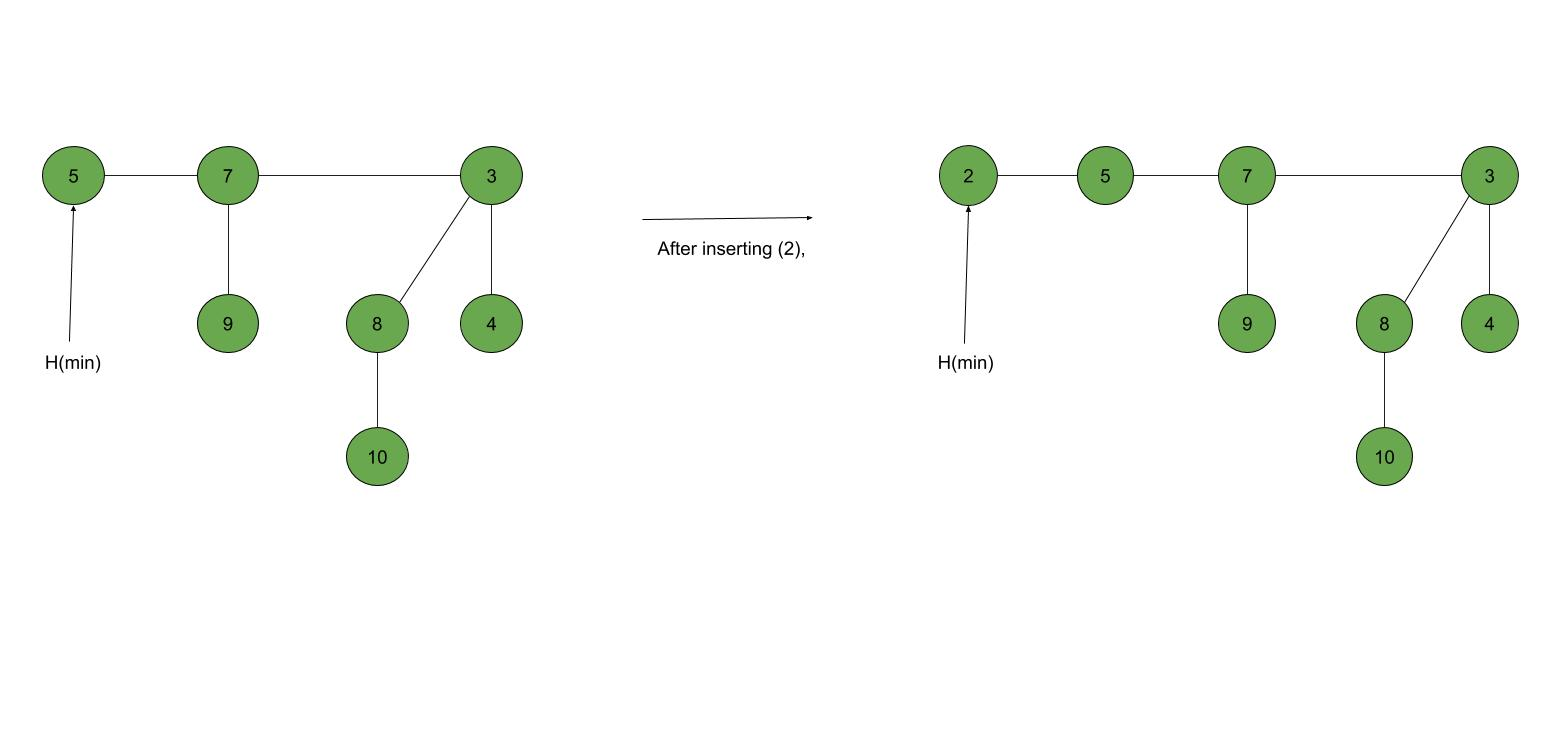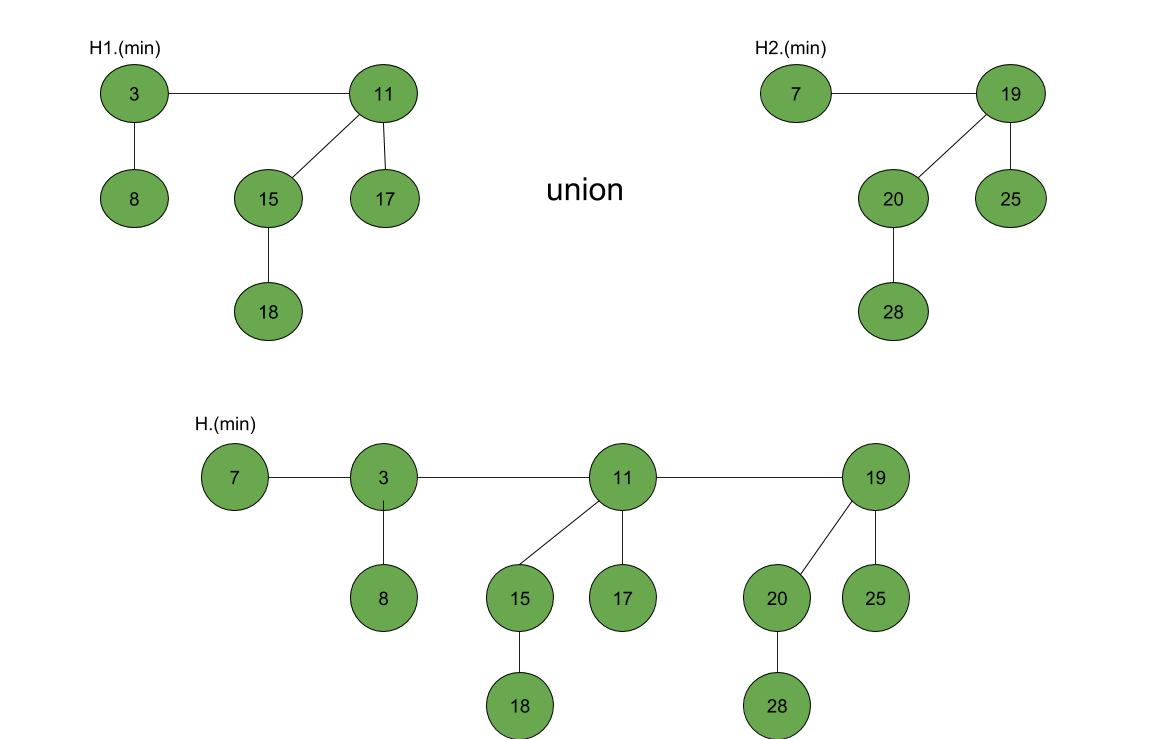Fibonacci Heap – Insertion and Union

• Difficulty Level : Medium
• Last Updated : 24 Nov, 2021

Prerequisites: Fibonacci Heap (Introduction)

Fibonacci Heap is a collection of trees with min-heap or max-heap property. In Fibonacci Heap, trees can have any shape even all trees can be single nodes (This is unlike Binomial Heap where every tree has to be Binomial Tree).
In this article, we will discuss Insertion and Union operation on Fibonacci Heap.

Insertion: To insert a node in a Fibonacci heap H, the following algorithm is followed:

1. Create a new node ‘x’.
2. Check whether heap H is empty or not.
3. If H is empty then:
• Make x as the only node in the root list.
• Set H(min) pointer to x.
4. Else:
• Insert x into root list and update H(min).

Example:Union: Union of two Fibonacci heaps H1 and H2 can be accomplished as follows:

1. Join root lists of Fibonacci heaps H1 and H2 and make a single Fibonacci heap H.
2. If H1(min) < H2(min) then:
• H(min) = H1(min).
3. Else:
• H(min) = H2(min).

Example:Following is a program to demonstrate building and inserting in a Fibonacci heap:

C++

 // C++ program to demonstrate building// and inserting in a Fibonacci heap#include #include #include using namespace std;  struct node {    node* parent;    node* child;    node* left;    node* right;    int key;};  // Creating min pointer as "mini"struct node* mini = NULL;  // Declare an integer for number of nodes in the heapint no_of_nodes = 0;  // Function to insert a node in heapvoid insertion(int val){    struct node* new_node = (struct node*)malloc(sizeof(struct node));    new_node->key = val;    new_node->parent = NULL;    new_node->child = NULL;    new_node->left = new_node;    new_node->right = new_node;    if (mini != NULL) {        (mini->left)->right = new_node;        new_node->right = mini;        new_node->left = mini->left;        mini->left = new_node;        if (new_node->key < mini->key)            mini = new_node;    }    else {        mini = new_node;    }}  // Function to display the heapvoid display(struct node* mini){    node* ptr = mini;    if (ptr == NULL)        cout << "The Heap is Empty" << endl;      else {        cout << "The root nodes of Heap are: " << endl;        do {            cout << ptr->key;            ptr = ptr->right;            if (ptr != mini) {                cout << "-->";            }        } while (ptr != mini && ptr->right != NULL);        cout << endl             << "The heap has " << no_of_nodes << " nodes" << endl;    }}// Function to find min node in the heapvoid find_min(struct node* mini){    cout << "min of heap is: " << mini->key << endl;}    // Driver codeint main(){      no_of_nodes = 7;    insertion(4);    insertion(3);    insertion(7);    insertion(5);    insertion(2);    insertion(1);    insertion(10);      display(mini);      find_min(mini);      return 0;}
Output:
The root nodes of Heap are:
1-->2-->3-->4-->7-->5-->10
The heap has 7 nodes
Min of heap is: 1

My Personal Notes arrow_drop_up